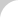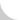The Complete Guide to Chain# 4.3 Selection Example

Now that we've covered the procedures you need to follow to choose a conveyor chain, let's complete an example.

Your assignment: Select a suitable chain for the conditions shown in Figure 4.23.

1. Operating conditions (Figure 4.23). In addition, note the following:
• The conveyed object is steel pipe supported on a plate.
• The system operates in a clean environment.
• The environment and conveyed objects are at ambient temperature.
• The chain can be lubricated.
3. The chain type: check both RF and RF-B types.
4. The roller type: R-roller.
5. The chain pitch: 250 mm.
6. The number of sprocket teeth: based on the chain pitch and the chain speed, select six teeth.
7. The attachment type: A-2.
8. Determine the chain size from the tension.

In this example, two sets of chain convey 80,000 kg. Therefore, each of the selected chains must be able to carry more than 40,000 kg per one set.

Table 4.5 shows you that either RF17 (general series) or RF10-B are acceptable.

Note: We are ignoring the dynamic tension of starting and stopping to make the example easier to understand.

Chain pitch is 250 mm and the length of the conveyed object is 1,000 mm.

Object length /pitch = the number of rollers under the conveyed object.

1,000 /250 = 4 rollers

If we use two sets of chain, there are eight rollers under one conveyed object. If the steel pipes on the plate are not carried equally, uneven load occurs on the roller. In this process, we presume that only four rollers share the load.

The roller's load = (2,000 × g) / 4 = 4,900 N = 4.9 kN

According to the catalog, either RF26 (standard series) or RF12-B (roller bearing) may be selected.Double Strand Conveyor
Conveyor Length: 50m
Chain Speed: 10m/min.
Weight of Conveyed Material: 2,000kg/pc × 40pcs
Chain: P=250mm  R-Roller  A-2 Attachment
Figure 4.23 Parameters for Example Selection Process

1. The allowable load of standard A attachment.
There are eight A attachments under each pallet. Assume that four attachments receive the load equally. The load on the A attachments = 4,900 N. According to the catalogs, RF12 (basic series) or stronger is acceptable.
2. Taking into account the tension, the allowable roller load, and the allowable load for standard A attachments, RF26250-R (general series) or RF12250-BR (roller bearing) may be selected.
3. Motor size.

Motor (kW) = (T × V) / 54.5 × (1 / η)  (η = 0.85 motor efficiency)

When using bearing roller conveyor chain, f1 = 0.03.

T = 2,000 kg × (g / 1,000) × 40 pieces × 0.03 = 23.5 kN {2,400 kgf}

kW = (23.5 × 10) / 54.5 × (1 / 0.85) = 5.1 kW

When using RF series conveyor chain, f1 = 0.08.

T = 2,000 kg × (g / 1,000) × 40 pieces × 0.08 = 62.8 kN {6,400 kgf}

kW = (62.8 × 10) / 54.5 × (1 / 0.85) = 13.6 kW

The process is straightforward and logical. And you can see that a bearing roller conveyor chain, because it has lower friction, allows you to use a smaller chain and a smaller motor.